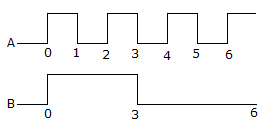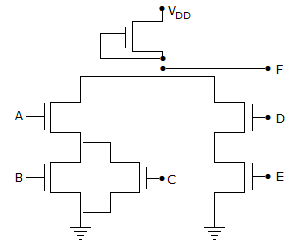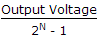# Electronics and Communication Engineering - Digital Electronics

21.

Assertion (A): Master slave JK flip flop is commonly used in high speed synchronous circuitry

Reason (R): Master slave JK flip flop uses two JK flip flops in cascade.

 A. Both A and R are correct and R is correct explanation of A B. Both A and R are correct but R is not correct explanation of A C. A is true, R is false D. A is false, R is true

Answer: Option D

Explanation:

R-S, J-K flip-flop is not used very commonly.

22.

Inputs A and B of the given figure are applied to a NAND gate. The output is LOWA. from 0 to 6 B. from 0 to 2 C. from 0 to 1 and 2 to 3 D. from 1 to 2 and 3 to 4

Answer: Option C

Explanation:

NAND gate gives Low output if all inputs are High. For other combinations of inputs, output is High.

23.

ECL is a saturating logic.

 A. True B. False

Answer: Option B

Explanation:

It is a non-saturating logic. Hence highest speed of operation.

24.

For the NMOS gate in the given figure, F =A. ABCDE B. (AB + C) (D + E) C. A(B + C) + DE D. A + B C + DE

Answer: Option C

Explanation:

B + C are in parallel and A is in series with this parallel combination, Similarly D + E are in series. Then D, E are in parallel with A, B and C Y = A(B + C) + DE .

25.

The resolution of 4 bit counting ADC is 0.5 volt, for an Analog input of 6.6 volts. The digital output of ADC will be

 A. 1011 B. 1101 C. 1100 D. 1110

Answer: Option B

Explanation:

Digital output of ADC resolution =.

#### Current Affairs 2021

Interview Questions and Answers